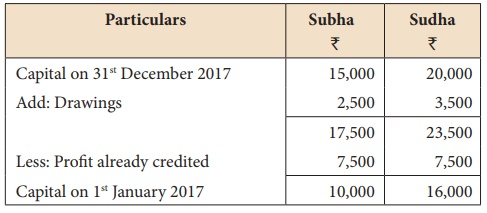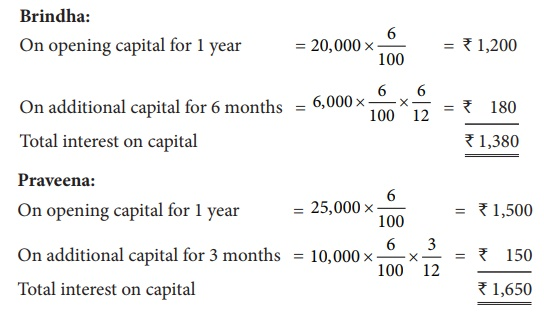Home | | Accountancy 12th Std | Calculation of interest on capital

# Calculation of interest on capital

Interest on capital is to be calculated on the capitals at the beginning for the relevant period.

Calculation of interest on capital

Interest on capital is to be calculated on the capitals at the beginning for the relevant period. If there is any additional capital introduced or capital withdrawn during the year, it will cause change in the capitals and interest is to be calculated proportionately on the changed capitals for the relevant period.

Interest on capital = Amount of capital x Rate of interest per annum x Period of interest

Tutorial note: If capital at the beginning is not given, then it can be calculated as below:Illustration 5

Mannan and Ramesh share profits and losses in the ratio of 3:1. The capital on 1st April 2017 was 80,000 for Mannan and 60,000 for Ramesh and their current accounts show a credit balance of 10,000 and 5,000 respectively. Calculate interest on capital at 5% p.a. for the year ending 31st March 2018 and show the journal entries.

Solution

Calculation of interest on capital:

Interest on capital = Amount of capital x Rate of interest

Interest on Mannan’s capital = 80,000 x 5/100 = 4,000

Interest on Ramesh’s capital = 60,000 x 5/100 = 3,000

Note: Balance of current account will not be considered for calculation of interest on capital.Illustration 6

Antony and Akbar were partners who share profits and losses in the ratio of 3:2. Balance in their capital account on 1st January 2018 was Antony 60,000 and Akbar 40,000. On 1st April 2018 Antony introduced additional capital of 10,000. Akbar introduced additional capital of 5,000 during the year. Calculate interest on capital at 6% p.a. for the year ending 31st December 2018.

SolutionNote: Since the date of additional capital introduced by Akbar is not given, interest on additional capital is calculated for an average period of 6 months.

Illustration 7

The capital account of Arivazhagan and Srinivasan on 1st January 2017 showed a balance of 15,000 and 10,000 respectively. On 1st July 2017, Arivazhagan introduced an additional capital of 5,000 and on 1st September 2017 Srinivasan introduced an additional capital of 10,000.

Calculate interest on capital at 6% p.a. for the year ending 31st December 2017.

SolutionIllustration 8

From the following balance sheets of Subha and Sudha who share profits and losses equally, calculate interest on capital at 6% p.a. for the year ending 31st December 2017.Drawings of Subha and Sudha during the year were ₹ 2,500 and ₹ 3,500 respectively. Profit earned during the year was ₹ 15,000.

SolutionCalculation of interest on capital:

Subha:

On opening capital = 10,000×[6/100] = ₹ 600

Sudha:

On opening capital = 16,000×[6/100] = ₹ 960

Illustration 9

From the following balance sheets of Brindha and Praveena who share profits and losses in the ratio of 3:4, calculate interest on capital at 6% p.a. for the year ending 31st December 2017.On 1st July 2017, Brindha introduced an additional capital of 6,000 and on 1st October 2017, Praveena introduced 10,000. Drawings of Brindha and Praveena during the year were 5,000 and 7,000 respectively. Profit earned during the year was 31,000.

Solution* Profit credited = Profit earned ₹ 31,000 – Balance profit as per balance sheet ₹ 10,000 = ₹ 21,000. This amount is distributed in their profit sharing ratio of 3:4.

Calculation of interest on capital:Illustration 10

A and B contribute 4,00,000 and 2,00,000 respectively as capital. Their respective share of profit is 3:2 and the profit before interest on capital for the year is 27,000. Compute the amount of interest on capital in each of the following situations:

(i) if the partnership deed is silent as to the interest on capital

(ii) if interest on capital @ 3% is allowed as per the partnership deed

(iii) if the partnership deed allows interest on capital @ 5% p.a.

Solution

(i) Interest on capital will not be allowed as the partnership deed is silent as to the interest on capital.

(ii) Profit before interest on capital is 27,000.

Computation of interest on capital:Since there is sufficient profit, interest on capital will be provided.

(iii) Profit before interest on capital is ₹ 27,000.

Computation of interest on capital:Since the profit is insufficient, interest on capital will not be provided. Profit of ₹ 27,000 will be distributed to the partners in their capital ratio of 2:1.

Tags : Accounts of Partnership Firms Fundamentals | Accountancy , 12th Accountancy : Chapter 3 : Accounts of Partnership Firms Fundamentals
Study Material, Lecturing Notes, Assignment, Reference, Wiki description explanation, brief detail
12th Accountancy : Chapter 3 : Accounts of Partnership Firms Fundamentals : Calculation of interest on capital | Accounts of Partnership Firms Fundamentals | Accountancy